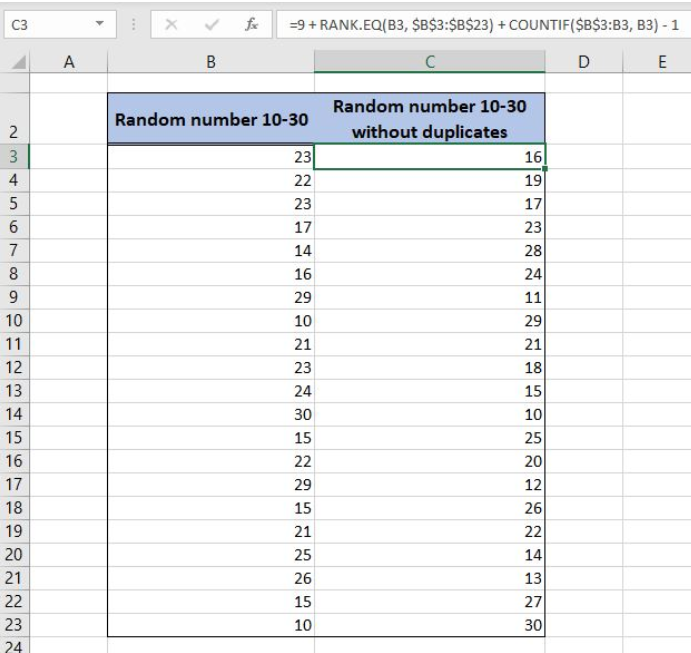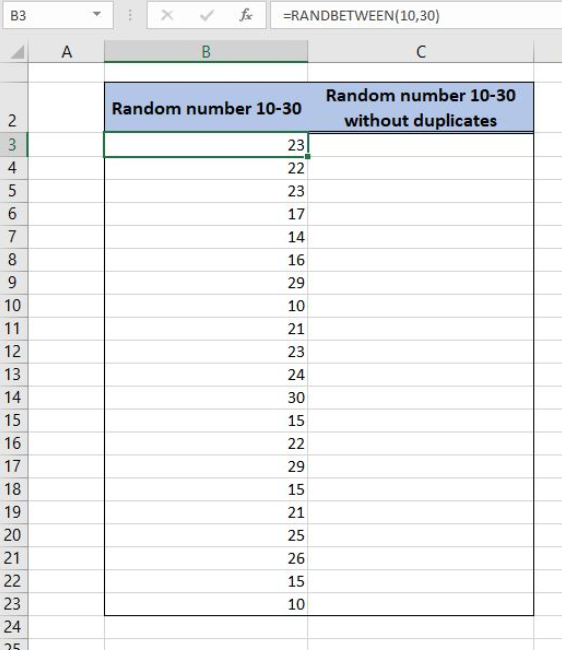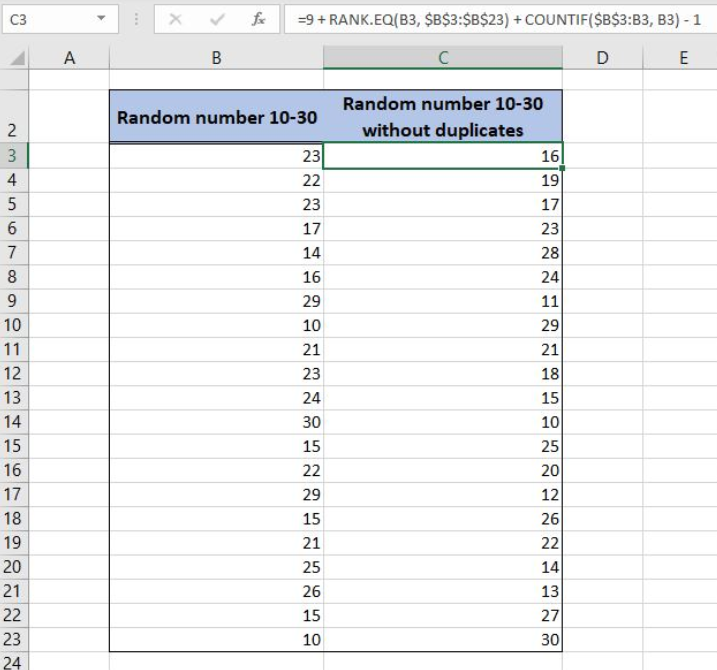Get instant live expert help with Excel or Google Sheets“My Excelchat expert helped me in less than 20 minutes, saving me what would have been 5 hours of work!”

#### Post your problem and you’ll get expert help in seconds.

Your message must be at least 40 characters
Our professional experts are available now. Your privacy is guaranteed.

# How to Use the RANDBETWEEN Function with No Duplicates

Excel allows us to create to generate random numbers list without duplicates using the RANDBETWEEN, RANK.EQ and COUNTIF functions. This step by step tutorial will assist all levels of Excel users to learn how to use the RANDBETWEEN function with no duplicates.Figure 1. The final result of the formula

## Syntax of the RANDBETWEEN Formula

`=RANDBETWEEN(bottom, top)`

The parameters of the RANDBETWEEN function are:

• bottom – a value from which we want to get a random value
• top – a value to which we want to get a random value

## Syntax of the RANK.EQ Formula

The generic formula for the RANK.EQ function is:

`=RANK.EQ(number, ref, [order])`

The parameters of the RANK.EQ function are:

• number – a number for which we want to find the rank in the array
• ref – the array where we want to rank the number
• [order] – a type of ranking data in the ascending or the descending order (0 or omitted value).

## Syntax of the COUNTIF Formula

The generic formula for the COUNTIF function is:

`=COUNTIF(range, criteria)`

The parameters of the COUNTIF function are:

• range – the range from which we want to count the non-blank cells
• criteria – the criteria for counting the cells in the range

## Generate Random Number List With No Duplicates in Excel

In column B, we will first generate random numbers from 10 and 30 using the RANDBETWEEN function. As the function can generate duplicate numbers, in column C, we will generate a new list of numbers without duplicates.

The formula in column B looks like:

`=RANDBETWEEN(10, 30)`

The bottom parameter of the function is 10, while the top parameter is 30.

To apply the RANDBETWEEN formula, we need to follow these steps:

• Select cell B3 and click on it
• Insert the formula: `=RANDBETWEEN(10,30)`
• Press enter
• Drag the formula down to the other cells in the column by clicking and dragging the little “+” icon at the bottom-right of the cell.Figure 2. Using the RANDBETWEEN formula to get random numbers 10-30 list

Now we can use the combination of RANK.EQ and COUNTIF functions to create a list without duplicates.

The formula in the cell C3 looks like:

`=9 + RANK.EQ(B3, \$B\$3:\$B\$23) + COUNTIF(\$B\$3:B3, B3) - 1`

At the beginning of the formula, we must add 9 to the result, as the range begins from 10. The parameter range in the COUNTIF function is the cell range \$B\$3:B3 while the criteria is the cell B3. The number parameter of the RANK.EQ function is the cell B3, while the parameter ref is the range \$B\$3:\$B\$23. An order is omitted because we want to rank in descending order.

To apply the formula, we need to follow these steps:

• Select cell C3 and click on it
• Insert the formula: `=9+RANK.EQ(B3,\$B\$3:\$B\$23)+COUNTIF(\$B\$3:B3,B3)-1`
• Press enter
• Drag the formula down to the other cells in the column by clicking and dragging the little “+” icon at the bottom-right of the cell.Figure 3. Generate a list of random numbers 10-30 with no duplicates

As we can see in Figure 3, column B has numbers 10-30 with duplicates, because only the RANDBETWEEN function is used. In column C, we have same numbers 10-30, but without duplicates using the RANK.EQ and COUNTIF functions.

Most of the time, the problem you will need to solve will be more complex than a simple application of a formula or function. If you want to save hours of research and frustration, try our live Excelchat service! Our Excel Experts are available 24/7 to answer any Excel question you may have. We guarantee a connection within 30 seconds and a customized solution within 20 minutes.

Solution examplesI need a formula to count the number of rows that contain at least one of two possible phrases, but not give me the total number of instances that both phrases occur overall.
Solved by E. B. in 33 minsi have a table with Dates, First names , Last names and i want to count how many times a name occurs, but if a name occurs more than once on a particular date i need to only count it once. this is on excel and not google sheets
Solved by F. H. in 31 minsNeed a formula that counts (1,2,3,4 ect..in cell M21) a range of cells, N4:N20, which are annual premium values. But I only want to add a sale count if the average monthly premium is above \$30 per sale. So if someone has 4 sales but they don't equal a total of \$120/mth (\$30 x 4 = \$120)... they would only get a count of 3 sales.
Solved by S. E. in 60 minsHello, I am trying to create a total color column? Can anyone help?
Solved by I. A. in 60 minsAny rep that has orders over 500 and occurrences at zero for each month, for a 6 month period. I need a sum
Solved by C. E. in 60 mins